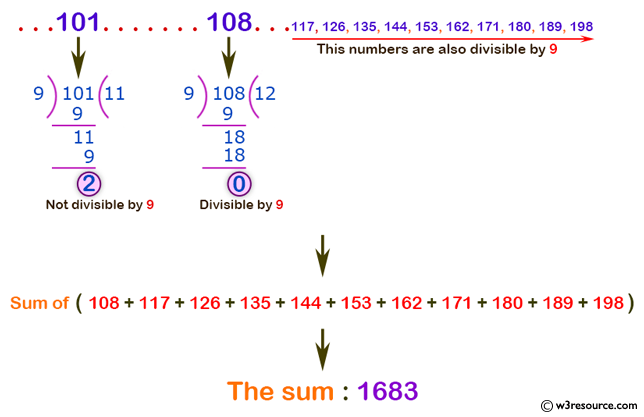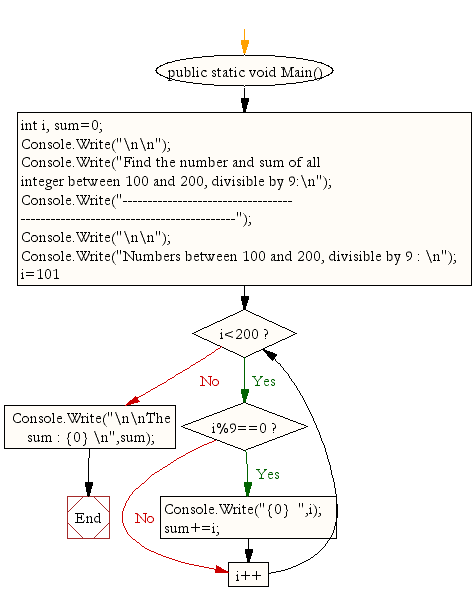﻿ C# - Count and sum all integers divisible by 9# C# Sharp Exercises: Find the number and sum of all integer between 100 and 200, divisible by 9

## C# Sharp For Loop: Exercise-39 with Solution

Write a program in C# Sharp to find the number and sum of all integer between 100 and 200 which are divisible by 9.

Pictorial Presentation:Sample Solution:

C# Sharp Code:

``````using System;
public class Exercise39
{
public static void Main()
{
int i, sum=0;

Console.Write("\n\n");
Console.Write("Find the number and sum of all integer between 100 and 200, divisible by 9:\n");
Console.Write("-----------------------------------------------------------------------------");
Console.Write("\n\n");

Console.Write("Numbers between 100 and 200, divisible by 9 : \n");
for(i=101;i<200;i++)
{
if(i%9==0)
{
Console.Write("{0}  ",i);
sum+=i;
}
}
Console.Write("\n\nThe sum : {0} \n",sum);
}
}
```
```

Sample Output:

```Find the number and sum of all integer between 100 and 200, divisible by 9:
-----------------------------------------------------------------------------
Numbers between 100 and 200, divisible by 9 :
108  117  126  135  144  153  162  171  180  189  198
The sum : 1683
```

Flowchart:C# Sharp Code Editor:

Contribute your code and comments through Disqus.

What is the difficulty level of this exercise?

Test your Programming skills with w3resource's quiz.

﻿